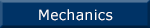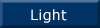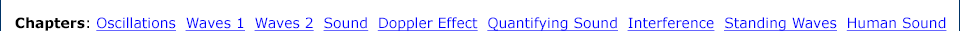Links to supporting pages and teachers' resources Background to Oscillations Inertia plus a restoring force produces oscillations. The mechanical equation for Simple Harmonic Motion. Initial conditions. Cyclic and angular frequency. Mechanical and kinetic energies. The simple pendulum. A nonlinear pendulum. Damped oscillations. Forced oscillations. Resonance in one, two and three dimensions. includesHelmholtz Oscillator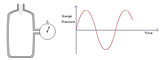Strings, standing waves and harmonics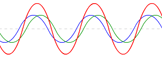Chladni patterns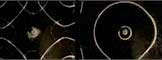Inertia and the second law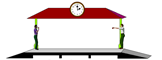Kinematics of Simple Harmonic Motion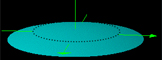Hooke's law and elasticity Revise calculus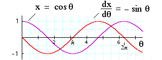Solving Differential Equations Methods for solving differential equations. First order with constant coefficients. Second order and simple harmonic motion. Damped and forced oscillation. Partial differential equations: the wave equation.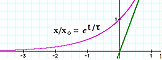Phasor addition Phasor representation of Simple Harmonic Motion. Adding phasors with different amplitudes and phase but equal frequency. Constructive and destructive interference. What if frequencies are different? Phasor and Lissajous representations compared.LaboratoryDownloads (thumbnails at 50% of size of animation)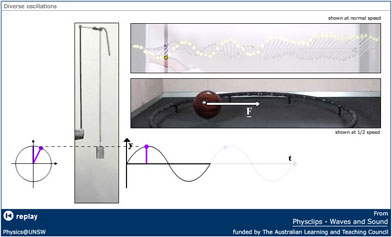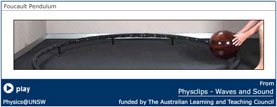Download (.zip) Download (.zip) Download (.zip)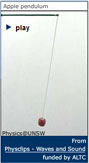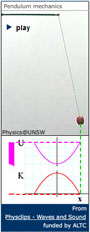Download (.zip) Download (.zip) Download (.zip)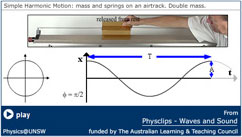Download (.zip) Download (.zip) Download (.zip)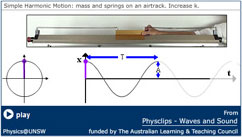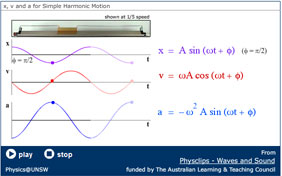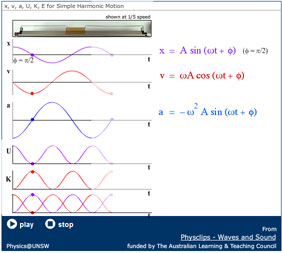Download (.zip) Download (.zip) Download (.zip)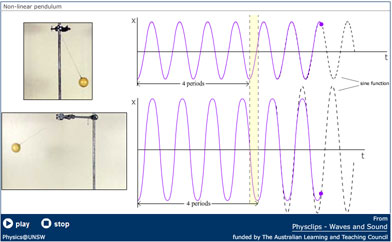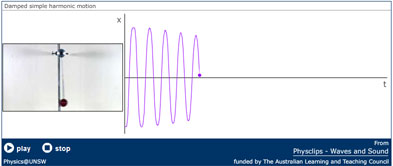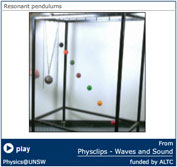Download (.zip) Download (.zip) Download (.zip)Download (.zip) Download (.zip)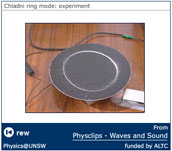Download (.zip) Download (.zip)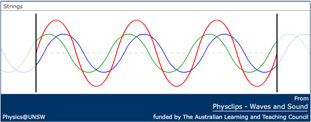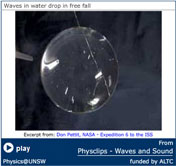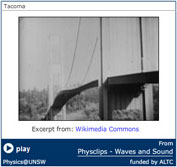Download (.zip) Download (.zip) Download (.zip)